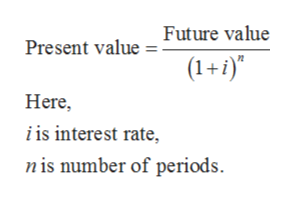# A couple plans to save for their child's college education. What principal must be deposited by the parents when their child is born in order to have \$43,000 when the child reaches the age of 18? Assume the money earns 7% interest, compounded quarterly. (Round your answer to two decimal places.)

Question
66 views

A couple plans to save for their child's college education. What principal must be deposited by the parents when their child is born in order to have \$43,000 when the child reaches the age of 18? Assume the money earns 7% interest, compounded quarterly. (Round your answer to two decimal places.)

check_circle

Step 1

Present value can be defined as the amount which is to be set aside in order to have a pre-defined sum at a future date at given interest rate.

It is given that,

Future value is \$43,000.

Interest rate is 7% compounded quarterly. So, interest rate per quarter will be (7% ÷ 4) = 1.75%.

Number of years is 18. So, total number of periods will be 18 × 4 = 72.

Step 2

The formula to calculate present value is given below:help_outlineImage TranscriptioncloseFuture value Present value (1+) Here, i is interest rate nis number of periods fullscreen
Step 3

Substitute \$43,000 for future value, 1.75% f...

### Want to see the full answer?

See Solution

#### Want to see this answer and more?

Solutions are written by subject experts who are available 24/7. Questions are typically answered within 1 hour.*

See Solution
*Response times may vary by subject and question.
Tagged in